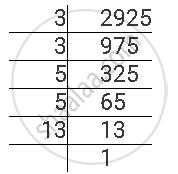Share

# For the Following Number, Find the Smallest Whole Number by Which It Should Be Divided So as to Get a Perfect Square Number. Also Find the Square Root of the Square Number So Obtained. 2925 - CBSE Class 8 - Mathematics

ConceptSquare Roots - Finding Square Root Through Prime Factorisation

#### Question

For the following number, find the smallest whole number by which it should be divided so as to get a perfect square number. Also find the square root of the square number so obtained.

2925

#### Solution

2925 can be factorised as follows.2925 = 3 x 3 x 5 x 5 x 13

Here, prime factor 13 does not have its pair.

If we divide this number by 13, then the number will become a perfect square. Therefore, 2925 has to be divided by 13 to obtain a perfect square.

2925 ÷13 = 225 is a perfect square.

225 = 3 x 3 x 5 x 5

∴ sqrt225 = 3 x 5 = 15

Is there an error in this question or solution?

#### APPEARS IN

NCERT Solution for Mathematics Textbook for Class 8 (2018 to Current)
Chapter 6: Squares and Square Roots
Ex. 6.30 | Q: 6.2 | Page no. 102
Solution For the Following Number, Find the Smallest Whole Number by Which It Should Be Divided So as to Get a Perfect Square Number. Also Find the Square Root of the Square Number So Obtained. 2925 Concept: Square Roots - Finding Square Root Through Prime Factorisation.
S